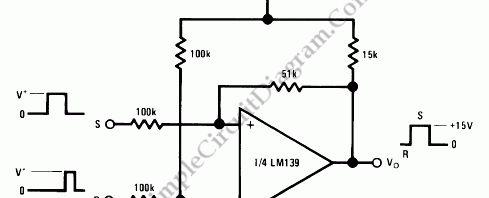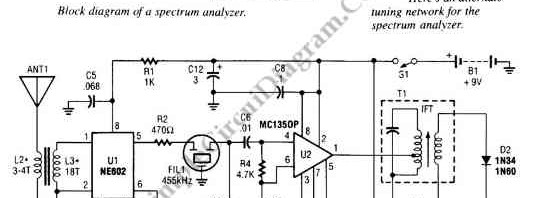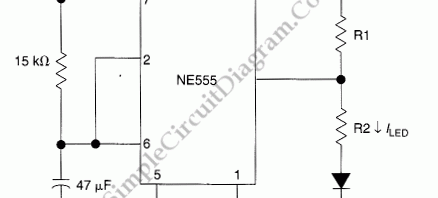## Bi-Stable Multivibrator (RS Flip-FLop) with Op-AmpThis bi-stable circuit uses an op-amp, acts similar with RS flip-flop. Bi-stable circuit is a circuit with two stable states, low and remain low until set is triggered, or high and remain high until reset is triggered. Here is the schematic diagram of the circuit:

## Amplitude Modulator with OTACircuit described in the schematic diagram below can achieve 99% modulation easily with maximum frequency of 200kHz. The amplification of this circuit can be controlled easily since the transconductance of an operational transconductance amplifier is directly propotional to the control current Iabc. The product from input voltage times the transconductance is the output current of this circuit. Here is the […]

## Spectrum Analyzer Adapter for OscilloscopesThe circuit shown in the following schematic diagram is a simple spectrum analyzer adapter circuit for oscilloscopes. This circuit can be used for scanning or monitoring a segment of radio spectrum or amateur band.  Inside this circuit,  an NE602 mixer chip generates a 455-kHz IF signal. The U2 is used to amplify the signal. Then the amplified signal is fed […]